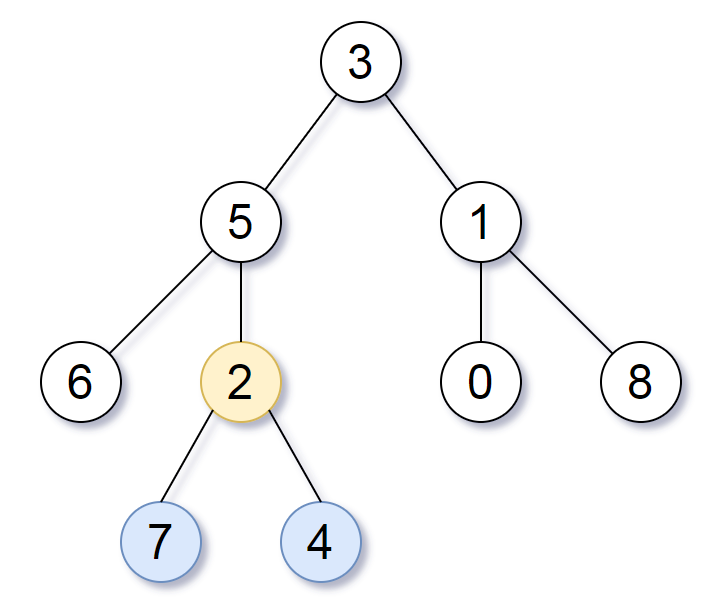# LeetCode Weekly Contest 92

https://leetcode.com/contest/weekly-contest-92

## Transpose Matrix

Given a matrix A, return the transpose of A.

The transpose of a matrix is the matrix flipped over it’s main diagonal, switching the row and column indices of the matrix.

Example 1:

Example 2:

Note:

1. 1 <= A.length <= 1000
2. 1 <= A.length <= 1000

## Smallest Subtree with all the Deepest Nodes

Given a binary tree rooted at root, the depth of each node is the shortest distance to the root.

A node is deepest if it has the largest depth possible.

Return the node with the largest depth such that it contains all the deepest nodes in it’s subtree.

Example 1:Note:

1. The number of nodes in the tree will be between 1 and 500.

1. 它的左右子树的高度相同
2. 它的高度与它父结点所表示的树只相差1

## Prime Palindrome

Find the smallest prime palindrome greater than or equal to N.

Recall that a number is prime if it’s only divisors are 1 and itself, and it is greater than 1.

For example, 2,3,5,7,11 and 13 are primes.

Recall that a number is a palindrome if it reads the same from left to right as it does from right to left.

For example, 12321 is a palindrome.

Example 1:

Example 2:

Example 3:

Note:

1. 1 <= N <= 10^8
2. The answer is guaranteed to exist and be less than 2 * 10^8.

1. 对于低位（对称的右半部分），如果该位值大于对称位的值，则向前进位，该位置为0
2. 把低位置为和对称位相等的值

## Shortest Path to Get All Keys

We are given a 2-dimensional grid. "." is an empty cell, "#" is a wall, "@" is the starting point, ("a", "b", …) are keys, and ("A", "B", …) are locks.

We start at the starting point, and one move consists of walking one space in one of the 4 cardinal directions. We cannot walk outside the grid, or walk into a wall. If we walk over a key, we pick it up. We can’t walk over a lock unless we have the corresponding key.

For some 1 <= K <= 6, there is exactly one lowercase and one uppercase letter of the first K letters of the English alphabet in the grid. This means that there is exactly one key for each lock, and one lock for each key; and also that the letters used to represent the keys and locks were chosen in the same order as the English alphabet.

Return the lowest number of moves to acquire all keys. If it’s impossible, return -1.

Example 1:

Example 2:

Note:

1. 1 <= grid.length <= 30
2. 1 <= grid.length <= 30
3. grid[i][j] contains only '.', '#', '@', 'a'-'f' and 'A'-'F'
The number of keys is in [1, 6]. Each key has a different letter and opens exactly one lock.